Custom SearchPROOF: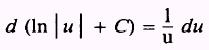Therefore,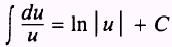NOTE: The derivative formula, presented in chapter 5, can be extended for negative functions also. Hence,EXAMPLE: Evaluate the integralSOLUTION: If we writewe find we are unable to evaluate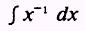by use of the power of a variable rule, so we writebecause the 1 dx in the numerator is precisely du and we have fulfilled the requirements for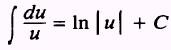EXAMPLE: Evaluate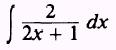SOLUTION: Letso that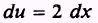We have the form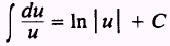therefore,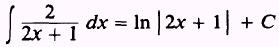EXAMPLE: Evaluate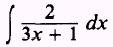SOLUTION: Let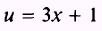so that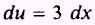We find we need 3 dx but we have 2 dx. We compensate as follows: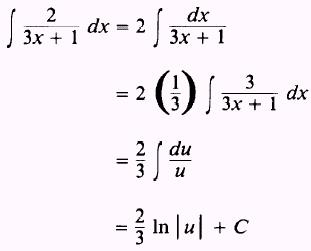Therefore,Integrated Publishing, Inc. - A (SDVOSB) Service Disabled Veteran Owned Small Business